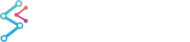﻿ CalculateRadiusToFit Method (ICameraController) | WPF Chart DocumentationExample
 SciChart.Charting3D Assembly > SciChart.Charting3D Namespace > ICameraController Interface : CalculateRadiusToFit Method
Calculates a Camera target and radius that will fit the bounding box completely inside the viewport. Uses a simple bounding-sphere algorithm
Syntax
```void CalculateRadiusToFit(
BoundingBox bounds,
out Vector3 newTarget,
)```

#### Parameters

bounds
newTarget
Example
```// Given a current position, target (which gives camera direction)
camera.Target = new Vector3(0,0,0);
camera.Position = new Vector3(100,100,100);

// Calculate optimum radius to fit the bounding box
Vector3 newTarget;